# How to Convert Pandas DataFrames to NumPy Arrays [+ Examples]Updated:

Published:

pandas is an open-source library built for fast and efficient manipulation of relational data in Python. It offers many built-in functions to cleanse and visualize data, but it is not as strong when it comes to statistical analysis. Fortunately, the NumPy library is also available in Python to dive deeper into the statistics of your data.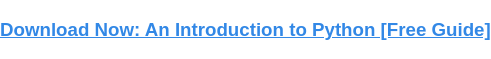NumPy is a library built for fast and complex statistical analysis. If you have your data captured in a pandas DataFrame, you must first convert it to a NumPy array before using any NumPy operations. Recognizing this need, pandas provides a built-in method to convert DataFrames to arrays: .to_numpy.

Before continuing, it's worth noting there are two alternative methods that are now discouraged: .as_matrix and .values. The first, .as_matrix, has been deprecated since pandas version 0.23.0 and will not work if called. The second, .values, is still supported but is discouraged in the pandas documentation in favor of .to_numpy. Keep this in mind when viewing older pandas files.

This post will cover everything you need to know to start using .to_numpy. Let's start by examining the basics of calling the method on a DataFrame.

## How to Convert Pandas DataFrames to NumPy Arrays

### Example 1: Convert DataFrame to NumPy array.

Here we'll review the base syntax of the .to_numpy method. To start, we have our existing DataFrame printed to the terminal below.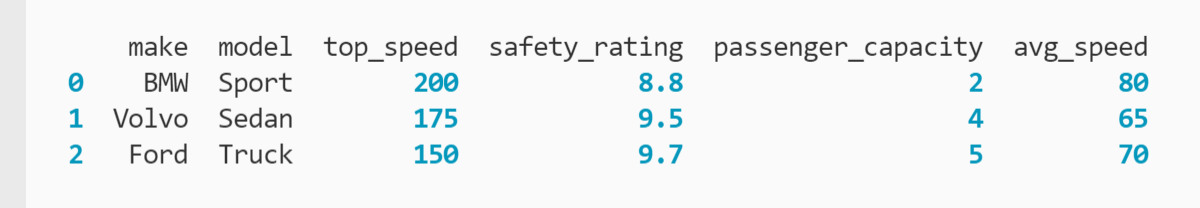To convert our DataFrame to a NumPy array, it's as simple as calling the .to_numpy method and storing the new array in a variable:

``` ```
car_arr = car_df.to_numpy()
``````

Here, car_df is the variable that holds the DataFrame. .to_numpy() is called to convert the DataFrame to an array, and car_arr is the new variable declared to reference the array.

We can confirm the method worked as expected by printing the new array to the terminal: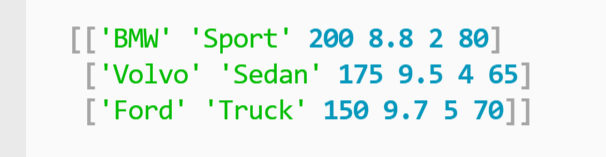Take a look at the structure of our new array. You can see that each row in our DataFrame is now a nested array within our parent array. This ensures that related values stay together.

Note that both NumPy arrays and Python Lists are denoted by the square brackets ([ ]). To confirm that .to_numpy created an array instead of a list, you can use the type function. These statements print both the array and its type to the terminal:

``` ```
print(car_arr)
print(type(car_arr))
``````

You can see the results of calling .to_numpy in the previous operation and the result of calling the type() function below.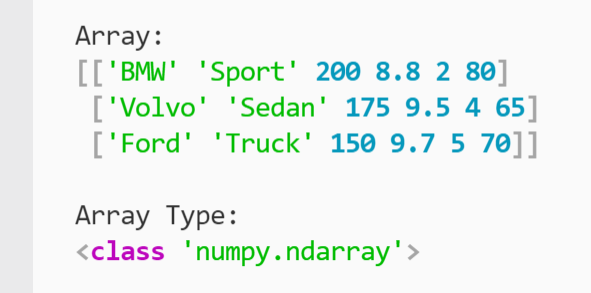You can now see that your DataFrame records are captured in an array structure and can confirm that it's a NumPy array.

Let's look at some more complex examples of converting pandas DataFrames to NumPy arrays.

### Example 2: Enforce data type and convert DataFrame to NumPy array.

Now we’ll start diving into the arguments available to us with .to_numpy to unlock more capabilities.

The first argument we'll inspect is data type. This value allows us to specify a data type for NumPy to apply to each of the values captured in the array.

For this example, we'll be using a new DataFrame that only contains integers and floats: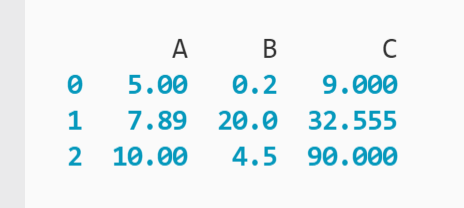Let's say you only wanted to store integers in your NumPy array. You can easily achieve this by declaring the data type in .to_numpy:

``` ```
num_arr = num_df.to_numpy(dtype = 'int')
``````

In this code, the dtype argument is set to "int" (short for integer). Printing the new num_arr variable to the terminal confirms the array only contains integers: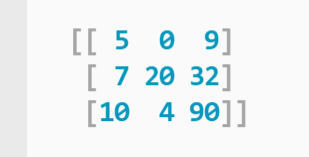You can see that NumPy does not perform any rounding. Instead, it simply removes anything after the decimal point in each value and leaves the base number. For example, 7.89 became 7.

If you want to preserve the decimal values, you can change dtype to "float." .to_numpy would most likely set the values to floats by default since there are already decimal values in the DataFrame, but this argument allows you to enforce that behavior against any edge cases.

Note that you need uniform data to properly implement data type. For example, if you tried to specify a float data type for a DataFrame that had rows containing strings, .to_numpy would fail and you would receive a ValueError. Instead, you would want to use the float data type when converting a DataFrame of numerical values to a NumPy array.

This is not to say you need to have a complete data set. .to_numpy provides you with a handy approach to handle null and missing values, as demonstrated in the next example.

### Example 3: Handle null values and convert DataFrame to NumPy array.

Let's return to the original DataFrame with our car model data. This time, however, it's missing a pair of values in the "avg_speed" column: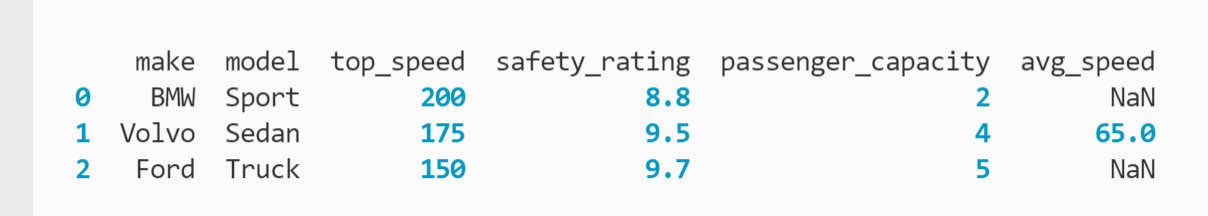Where we should have the average speeds for the first and third rows, instead we have NaN (not a number) markers. In other words, these are null values. Rather than persisting these values into our NumPy array, we can tell .to_numpy to handle them for us:

``` ```
car_arr = car_df.to_numpy(na_value = 50)
``````

Here, we use the na_value argument to tell NumPy we want any null values set to the base value 50. The average speed values are now updated accordingly in our NumPy array: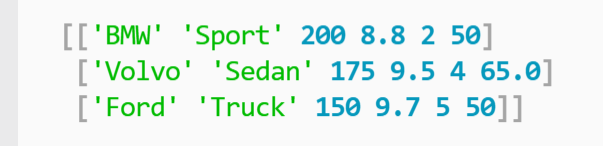Whether it's better to leave null values in place or replace them is determined by the parameters of your data analysis and the data governance policies in your organization.

Since this data deals with individual car attributes, it may be better to leave the null values in so that other data engineers know the data quality of the average speed set of values is not reliable and they won't draw false conclusions. In contrast, a large data set may be more tolerant of a few missing or placeholder values because they are less likely to affect calculations that involve all rows.

Also note that if you had null values in multiple columns (e.g. "make," "top_speed," and "avg_speed"), the na_value argument will be applied universally, so it's not always the best to use when converting full DataFrames. Otherwise, we could end up with 50 for the name of a carmaker in this example.

These considerations mean that the na_value argument is best used when converting individual DataFrame columns to arrays instead of the entire DataFrame. We'll review that syntax next.

### Example 4: Convert individual DataFrame columns to NumPy arrays.

A natural use case for NumPy arrays is to store the values of a single column (also known as a Series) in a pandas DataFrame. We can achieve this by using the indexing operator and .to_numpy together:

``` ```
car_arr = car_df['avg_speed'].to_numpy()
``````

Here, we are using the indexing operator ([ ]) to search for the index label "avg_speed" within the DataFrame. Once it finds the referenced column, .to_numpy() converts the column data into an array:To return to the last example, we can now deploy the na_value argument to replace missing and null values in a more limited scope:

``` ```
car_arr = car_df['avg_speed'].to_numpy(na_value = 50)
``````

Now we are no longer risking our replacement value being added to columns where it doesn't make sense.

## Optimize analysis by converting your Pandas DataFrame to NumPy arrays.

pandas is a powerful library for handling relational data, but like any code package, it's not perfect in every use case. NumPy is a second library built to support statistical analysis at scale. By converting your pandas DataFrames to NumPy arrays, you can enjoy the benefits of both frameworks while optimizing your data storage and analysis.Topics: What Is Python?

## Related Articles

We're committed to your privacy. HubSpot uses the information you provide to us to contact you about our relevant content, products, and services. You may unsubscribe from these communications at any time. For more information, check out our Privacy Policy.

A guide for marketers, developers, and data analysts.